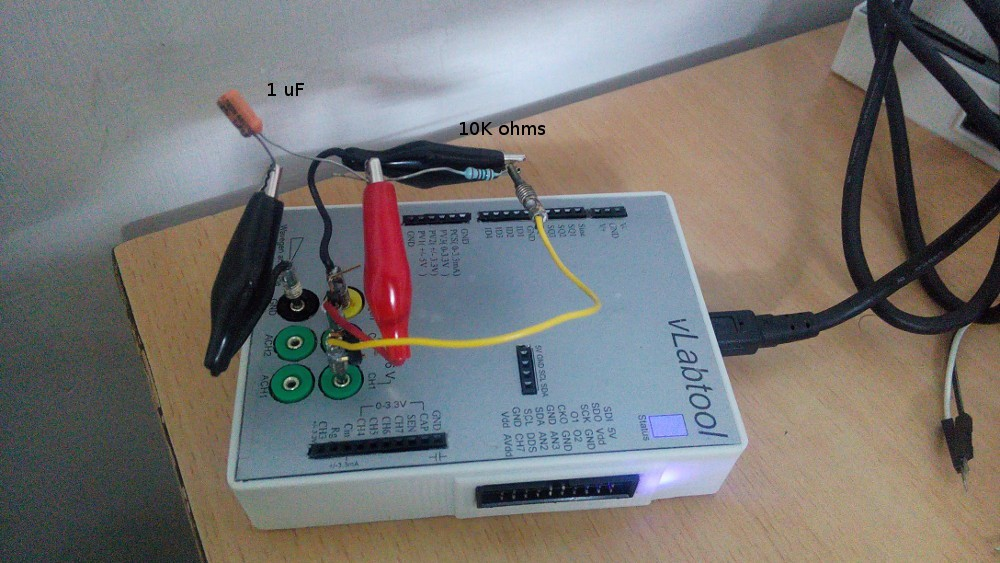# Basic Electronics Experiments• Characterize an RC filter and plot its frequency response and phase shifts.
• Measure the IV characteristics of a 4148 diode, and plot the dependence of the threshold voltage on temperature.
• A simple low-pass RC filter was made with a 10K resistor and 1uF capacitor. The input was taken from the programmable sine wave generator, and simultaneously monitored via CH1 . The output(midpoint of R and C) was also monitored via CH2.
Frequency was swept from 10Hz to 500Hz, and the amplitude and phase plots are shown

Photograph of the setup:• A 4148 diode’s IV characteristics are plotted.

Input is taken from a 0-3.3V DAC (PVS3) , and fed to a 4148 diode via a 1K resistor. The other end of the diode is grounded via a current to voltage convertor.

IV plots are obtained by plotting the voltage across the diode versus the current

• Set the base current using the constant current source.
sweep the collector voltage, and simultaneously monitor I_ce